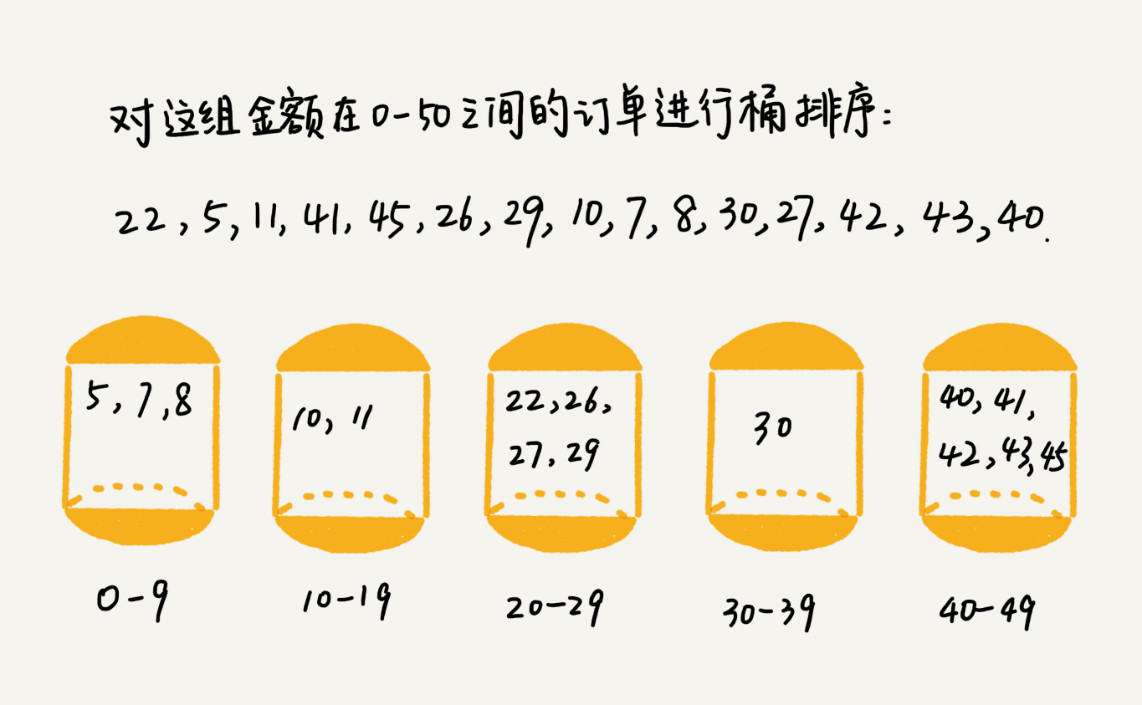# 如何把百万级别的订单根据金额排序「码哥」带大家学习的算法类比「超跑型赛车」，用于特殊场景能实现飞快速度。快排、归并是经济实用型小轿车。而桶排序、计数排序、基数排序则是赛道上的跑车竞速，也叫做线性排序

## 桶排序（Bucket Sort）

1. 在额外空间充足的情况下，尽量增加桶的数量。

2. 使用的映射函数能够把输入的 n 个数据均匀的分配到 k 个桶中。1. 要排序的数据很容易均匀的划分成 k 个桶，并且桶与桶之间有着天然的大小顺序。这样才在实现在每个桶内的数据都排序好了以后不需要再进行排序。

2. 数据在每个桶之间都是均匀的分布，假如出现有的桶很多数有的很少。那桶内排序的时间复杂度就不是常量级了，在极端情况下数据都划分到一个桶里，也就退化成 O(nlogn) 的时间复杂度了。

### 代码实战

/** * 桶排序：把数组 arr 划分为n个大小相同子区间（桶），每个子区间各自排序，最后合并 */public class BucketSort implements LineSort {    private static final QuickSort quickSort = new QuickSort();    @Override    public int[] sort(int[] sourceArray, int bucketSize) {        // 找出最大、最小值        int minValue = sourceArray;        int maxValue = sourceArray;        for (int value : sourceArray) {            minValue = Math.min(minValue, value);            maxValue = Math.max(maxValue, value);        }        // 桶数量        int bucketCount = (maxValue - minValue) / bucketSize + 1;        int[][] buckets = new int[bucketCount][bucketSize];        // 保存每个桶的数组的元素下标，默认值 0        int[] indexArr = new int[bucketCount];        // 将数组中值分配到各个桶里        for (int value : sourceArray) {            int bucketIndex = (value - minValue) / bucketSize;            // 当前桶的数组达到最大值,需要拓容            if (indexArr[bucketIndex] == buckets[bucketIndex].length) {                ensureCapacity(buckets, bucketIndex);            }            // 将数据放到桶中，并且桶对应的数组下标 + 1            buckets[bucketIndex][indexArr[bucketIndex]++] = value;        }        // 对每个桶进行排序，这里使用了快速排序        int k = 0;        for (int i = 0; i < buckets.length; i++) {            if (indexArr[i] == 0) {                continue;            }            // 默认容量是 bucketSize, 要根据实际桶的容量排序，否则不足 bucketSize 的默认值是 0            quickSort.quickSortInternal(buckets[i], 0, indexArr[i] - 1);            for (int j = 0; j < indexArr[i]; j++) {                sourceArray[k++] = buckets[i][j];            }        }        return sourceArray;    }    /**     * 数组扩容,并保存数据     *     * @param buckets     * @param bucketIndex     */    private void ensureCapacity(int[][] buckets, int bucketIndex) {        int[] tempArr = buckets[bucketIndex];        int[] newArr = new int[tempArr.length * 2];        for (int j = 0; j < tempArr.length; j++) {            newArr[j] = tempArr[j];        }        buckets[bucketIndex] = newArr;    }}

### 单元测试

@DisplayName("线性排序算法测试")public class LineSortTest {    private static int length = 100;    private int[] array = new int[length];    @BeforeEach    public void beforeEach() {        Random rand = new Random();        for (int i = 0; i < length; i++) {            // 随机生成 [1, 1000000] 的数据            array[i] = rand.nextInt(length) + 1;        }    }    @DisplayName("桶排序")    @Test    public void testBucketSort() {        BucketSort bucketSort = new BucketSort();        // 100 数据，10 个桶        int[] sort = bucketSort.sort(array, 10);        System.out.println(Arrays.toString(sort));    }}

## 总结### 码哥字节

Java 技术栈知识分享，关注公号：码哥字节 2019.06.10 加入

## 评论